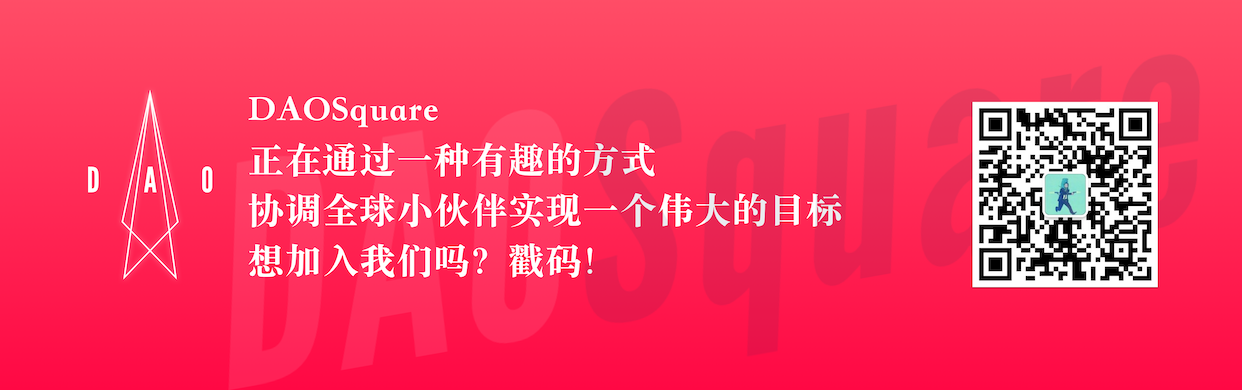### DAO是什么呢，莫奇？

—— Typto

​翻译机构：DAOSquare“我亲爱的小莫奇，”QwwQ说道，“人人都知道它带着个'T'字儿的。”

(QwwQ) <

(n_ll) <

(QwwQ) <

(n_ll) <

(QwwQ) <

(n_ll) <

(QwwQ) <

(n_ll) <

(QwwQ) <

(n_ll) <

(QwwQ) <

(n_ll) <

(QwwQ) <

(n_ll) <

(QwwQ) <

(n_ll) <

(QwwQ) <

(n_ll) <

(QwwQ) <

(n_ll) <

(QwwQ) <

(n_ll) <

(QwwQ) <

(n_ll) <

(QwwQ) <

(n_ll) <

🤦‍♀️不是啦，QwwQ.钱财并不是针对组织性问题的答案。再猜猜看。

(QwwQ) <

(n_ll) <

(QwwQ) <

(n_ll) <

？？？（疑惑）

(QwwQ) <

(n_ll) <

(QwwQ) <

(‘n.ll’) <

(‘QwwQ’) <

(n_ll) <

(‘QwwQ) <

(n_ll) <

(‘QwwQ) <

(n_ll) <

(‘QwwQ) <

(n_ll) <

...有的关于DAO用途的误读，他们认为DAO只是造成了金钱的流动，而任何一人都可以成为领导者。但其实，我们更致力于研究的是，我们应该如何让人们同样自由的流动，而无需领导者的发号施令。

(‘-ww-) <

(n_ll) <

(QwwQ) <

(QwwQ) <

...

(QwwQ) <

(n_ll) <

(QwwQ) <

(n_ll) <

(‘QwwQ) <• 发表于 2020-04-21 11:52
• 阅读 ( 468 )
• 学分 ( 8 )
• 分类：资讯